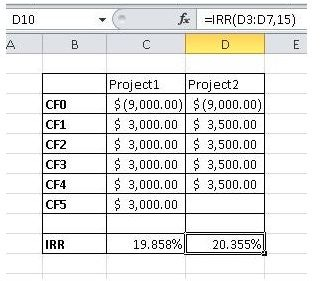# Calculating the Internal Rate of Return as a Capital Budgeting Tool

Page content

## What is a Project’s Internal Rate of Return?

The internal rate of return (IRR) is a capital budgeting term used to compare projects and to select the ones that offer the most benefit

(or return) for given capital expenditures. Simply put, the IRR is the discount rate that is required to make the present value of the project’s cost equal the present value of the project’s future cash inflows.

Determination of projects that should be accepted hinges on the IRR being greater than the rate of return that is required by an organization. Of course, sometimes different projects with similar associated risks may both exceed the so-called hurdle rate, in which case the project with the higher IRR will normally be accepted.

Often, companies will require that proposed projects come with a calculation of their IRR so that they only consider projects that meet their rate of return requirements.

## The Basic Formula for Calculating IRR

The calculation of the internal rate of return begins with considering cash inflows for a project over time compared to the initial cost. For each year the net present value for incoming cash must be calculated and added to the initial cash flow to equal zero. The equation to use looks like this:

NPV=0= CF0 + CF1/(1+r)^1+CF2/(1+r)^2+CF3/(1+r)^3…..

Estimating the value of r is possible using a complicated formula, but it’s a lot easier to use a business calculator such as the HP BA II or a spreadsheet program such as Microsoft Excel. You can use the IRR as part of the construction of a functional requirements matrix.

## An Example of Comparing IRR between Projects

To help you understand the process of calculating internal rate of return, let’s consider an example. A project requiring an expenditure of \$9,000 is expected to produce incoming cash flows of \$3,000 per year for five years with a 10% discount rate. A second project requires a \$9,000 investment and then will produce sales of \$3,500 per year for four years and a 15% discount rate. Let’s punch this into a spreadsheet and you will get the following results. The formula for the IRR is =IRR(C3:C8,10) for Project1 and =IRR(D3:D7,15) for Project 2.By these calculations, Project 2 would be selected since it has a higher IRR.

## Conclusion: IRR and Beyond

Now that you know how to calculate project internal rate of return, you should be reminded that the use of IRR to determine the acceptance of a project is not foolproof. This is primarily caused by the possibility of multiple IRRs for a project and the problem with its reinvestment rate assumption that overlooks which project best utilizes IRR. Also, a modified internal rate of return can be calculated that assumes that cash flows from a project are re-invested at the company’s cost of capital. Although we do not address these issues here, knowing that there are problems with IRR will suffice for now.

When deciding between two projects for capital investment, comparing internal rates of return can provide useful information for decision making.